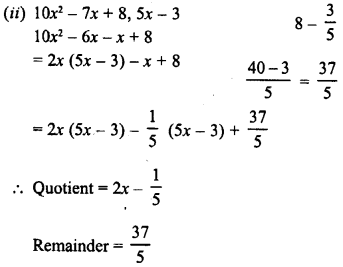## RD Sharma Class 8 Solutions Chapter 8 Division of Algebraic Expressions Ex 8.5

These Solutions are part of RD Sharma Class 8 Solutions. Here we have given RD Sharma Class 8 Solutions Chapter 8 Division of Algebraic Expressions Ex 8.5

Other Exercises

Question 1.
Divide the first polynomial by the second polynomial in each of the following. Also, write the quotient and remainder.
(i) 3x2 + 4x + 5, x – 2
(ii) 10x2 – 7x + 8, 5x – 3
(iii) 5y3– 6y2 + 6y-1,5y-1
(iv)x4-x3 + 5x,x-1
(v) y4 +y2,y2-2
Solution:
(i) 3x2 + 4x + 5, x – 2
= 3x (x – 2) + 10x + 5
= 3x (x – 2) + 10 (x – 2) + 25
∴ Quotient = 3x + 10
Remainder = 25(iii) 5y3 – 6y2 + 6y – 1, 5y – 1
= y(5y – 1) – 5y2 + 6y- 1
= y2 (5y – 1) -y (5y – 1) + 5y – 1
= y2 (5y- 1) -y (5y- 1) + 1 (5y- 1)
∴ Quotient = y2 – y + 1 and Remainder = 0
(iv) x4 – x3 + 5x, x – 1
= x3(x – 1) + 5x
= x3 (x – 1) + 5 (x – 1) + 5
∴ Quotient = x3 + 5, Remainder = 5
(v) y4+y2,y2– 2
= y2(y– 2) + 3y2
= y2 (y2 – 2) + 3 (y2 – 2) + 6
∴ Quotient =y2 + 3 and Remainder = 6

Question 2.
Find, whether or not the first polynomial is a factor of the second :
(i) x + 1, 2x2 + 5x + 4
(ii) y- 2, 3y3 + 5y2 + 5y + 2
(iii) 4x2 – 5, 4.x4 + 7x2 + 15
(iv) 4-z, 3z2 – 13z + 4
(v) 2a-3,10a2 – 9a – 5
(vi) 4y+1 ,8y2-2y + 1
Solution:
(i) x + 1, 2x2 + 5x + 4
2x2 + 5x + 4 = 2x (x + 1) + 3x + 4
= 2x (x + 1) + 3 (x + 1) + 1
∵ Remainder = 1
∴ x + 1 is not a factor of 2x2 + 5x + 4
(ii) y – 2, 3y3 + 5y2 + 5y + 2
3y3 + 5y2 + 5y + 2 = 3y2(y – 2)+11y2 + 5y + 2
= 3y2(y – 2)+11y (y – 2) + 27y + 2
= 3y2 (y – 2) + 11y (y – 2) + 27 (y – 2) + 56
∵ Remainder = 56
∴ y – 2 is not a factor of 3y3 + 5y2 + 5y + 2
(iii) 4x2 – 5, 4x4 + 7x2 + 15
4x4 + 7x2 + 15 = x2 (4x2 – 5) + 12x2 + 15
= x2 (4x2 – 5) + 3 (4x2 – 5) + 30
∵ Remainder = 30
∴ 4x2 – 5 is not a factor of 4x4 + 7x2 + 15
(iv) 4 – z, 3z2 – 13z + 4
3z2 – 13z + 4 = -3z (-z + 4) – z + 4
= -3z (-z + 4) + 1 (-z + 4)
∵ Remainder = 0
∴ 4 – z or – z + 4 is a factor of 3z2 – 13z + 4
(v) 2a – 3, 10a2 – 9a – 5
10a2 – 9a – 5 = 5a (2a – 3) + 6a – 5
= 5a (2a – 3) + 3 (2a – 3) + 4
∵ Remainder = 4
∴ 2a – 3 is not a factor of 10a2 – 9a – 5
(vi) 4y + 1, 8y2 – 2y + 1
8y2 – 2y + 1 = 2y (4y + 1) – 4y + 1
= 2y (4y + 1) – 1 (4y + 1) + 2
∵ Remainder = 2
∴ 4y + 1 is not a factor of 8y2 – 2y + 1

Hope given RD Sharma Class 8 Solutions Chapter 8 Division of Algebraic Expressions Ex 8.5 are helpful to complete your math homework.

If you have any doubts, please comment below. Learn Insta try to provide online math tutoring for you.